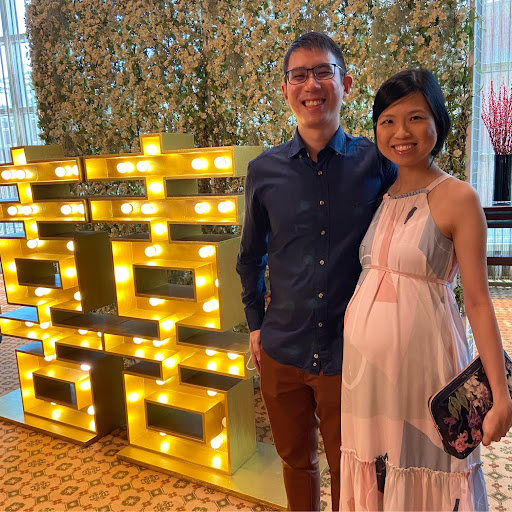tlhy -3 years ago 207
Python Question

# Django Tutorial - choice_set typeerror

So I was looking at this post to try and resolve some issues encountered in my Django tutorial:

TypeError: 'choice_text' is an invalid keyword argument for this function in django tutorial

this is my code:

``````from polls.models import Question, Choice
``````

I'm still facing exactly the same problem after taking the suggested solution of changing "choice_text" to "choice" - i.e exactly the same error message, and the documentation version for the Django tutorial is for Django 1.11 (my version). Does anybody know the correct syntax for creating choice sets?

Thanks!

Supplementary Information: My models.py file defining Question and Choice.

``````class Question(models.Model):
question_text = models.CharField(max_length=200)
pub_date = models.DateTimeField('date published')

def __str__(self):
return self.question_text

def was_published_recently(self):
return self.pub_date >= timezone.now() - datetime.timedelta(days=1)

class Choice(models.Model):
choice_texct = models.CharField(max_length=200)

def __str__(self):
return self.choice_text
``````Bear Brown

You have a typo in model:

``````choice_texct = models.CharField(max_length=200)
#      ^^^^
``````

need replace

``````choice_text = models.CharField(max_length=200)
#      ^^^^
``````

and don't forget do migrations,

``````q.choice_set.create(choice_text='Not much', votes=0)
``````q.choice_set.create(choice_texct='Not much', votes=0)